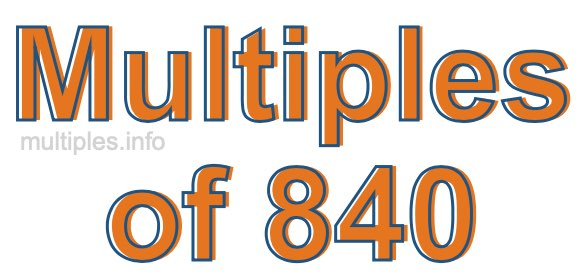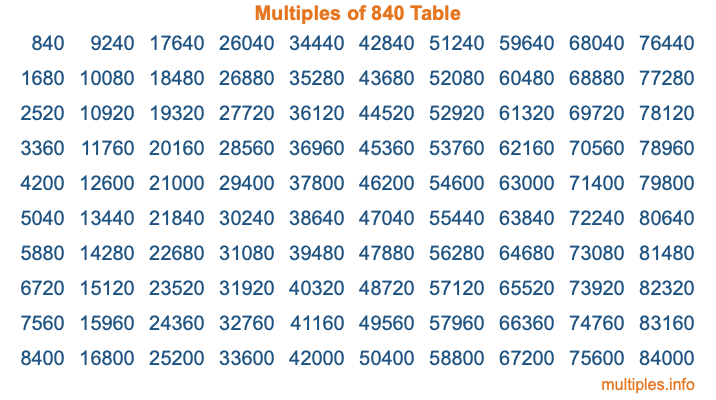Multiples of 840Welcome to the Multiples of 840 page. Here we will first teach you everything you will ever need to know about the multiples of 840, and then give you a study guide summary of everything we taught you to make sure you remember it all. Use this page to look up facts and learn information about the multiples of 840. This page will make you a multiples of eight hundred forty expert!

Definition of Multiples of 840
Multiples of 840 are all the numbers that when divided by 840 equal an integer. Each of the multiples of 840 are called a multiple. A multiple of 840 is created by multiplying 840 by an integer.

Therefore, to create a list of multiples of 840, you start with 1 multiplied by 840, then 2 multiplied by 840, then 3 multiplied by 840, and so on for as long as you want. Thus, the list of the first five multiples of 840 is 840, 1680, 2520, 3360, and 4200. To see a larger list of multiples of 840, see the printable image of Multiples of 840 further down on this page. We also have a category where you can choose any nth multiple of 840.

Multiples of 840 Checker
The Multiples of 840 Checker below checks to see if any number of your choice is a multiple of 840. In other words, it checks to see if there is any number (integer) that when multiplied by 840 will equal your number. To do that, we divide your number by 840. If the the quotient is an integer, then your number is a multiple of 840.

Is  a multiple of 840?

Least Common Multiple of 840 and ...
A Least Common Multiple (LCM) is the lowest multiple that two or more numbers have in common. This is also called the smallest common multiple or lowest common multiple and is useful to know when you are adding our subtracting fractions. Enter one or more numbers below (840 is already entered) to find the LCM.

Check out our LCM Calculator if you need more details about the Least Common Multiple or if you need the LCM for different numbers for adding and subtraction fractions.

nth Multiple of 840
As we stated above, 840 is the first multiple of 840, 1680 is the second multiple of 840, 2520 is the third multiple of 840, and so on. Enter a number below to find the nth multiple of 840.

th multiple of 840

Multiples of 840 vs Factors of 840
840 is a multiple of 840 and a factor of 840, but that is where the similarities end. All postive multiples of 840 are 840 or greater than 840. All positive factors of 840 are 840 or less than 840.

Below is the beginning list of multiples of 840 and the factors of 840 so you can compare:

Multiples of 840: 840, 1680, 2520, 3360, 4200, etc.

Factors of 840: 1, 2, 3, 4, 5, 6, 7, 8, 10, 12, 14, 15, 20, 21, 24, 28, 30, 35, 40, 42, 56, 60, 70, 84, 105, 120, 140, 168, 210, 280, 420, 840

As you can see, the multiples of 840 are all the numbers that you can divide by 840 to get a whole number. The factors of 840, on the other hand, are all the whole numbers that you can multiply by another whole number to get 840.

It's also interesting to note that if a number (x) is a factor of 840, then 840 will also be a multiple of that number (x).

Multiples of 840 vs Divisors of 840
The divisors of 840 are all the integers that 840 can be divided by evenly. Below is a list of the divisors of 840.

Divisors of 840: 1, 2, 3, 4, 5, 6, 7, 8, 10, 12, 14, 15, 20, 21, 24, 28, 30, 35, 40, 42, 56, 60, 70, 84, 105, 120, 140, 168, 210, 280, 420, 840

The interesting thing to note here is that if you take any multiple of 840 and divide it by a divisor of 840, you will see that the quotient is an integer.

Multiples of 840 Table
Below is an image of the first 100 multiples of 840 in a table. The table is in chronological order, column by column. The first column has the first ten multiples of 840, the second column has the next ten multiples of 840, and so on.The Multiples of 840 Table is also referred to as the 840 Times Table or Times Table of 840. You are welcome to print out our table for your studies.

Negative Multiples of 840
Although not often discussed or needed in math, it is worth mentioning that you can make a list of negative multiples of 840 by multiplying 840 by -1, then by -2, then by -3, and so on, to get the following list of negative multiples of 840:

-840, -1680, -2520, -3360, -4200, etc.

Multiples of 840 Summary
Below is a summary of important Multiples of 840 facts that we have discussed on this page. To retain the knowledge on this page, we recommend that you read through the summary and explain to yourself or a study partner why they hold true.

There are an infinite number of multiples of 840.

A multiple of 840 divided by 840 will equal a whole number.

840 divided by a factor of 840 equals a divisor of 840.

The nth multiple of 840 is n times 840.

The largest factor of 840 is equal to the first positive multiple of 840.

840 is a multiple of every factor of 840.

840 is a multiple of 840.

A multiple of 840 divided by a divisor of 840 equals an integer.

840 divided by a divisor of 840 equals a factor of 840.

Any integer times 840 will equal a multiple of 840.

Multiples of a Number
Here you can get the multiples of another number, all with the same attention to detail as we did for multiples of 840 on this page.

Multiples of
Multiples of 841
Did you find our page about multiples of eight hundred forty educational? Do you want more knowledge? Check out the multiples of the next number on our list!